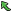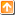## 3944: Maze, amazingTime Limit(Common/Java):1000MS/3000MS     Memory Limit:65536KByte
Total Submit: 20            Accepted:3

Description

Let’s play maze game again! Maze problem is an old problem but here comes a challenge one. Max, an intelligent robot, is trapped in an N*M maze grid. He is located at a start position initially and aiming to find the exit of the maze. In the maze there are numbers of pillars which are set as obstacles. Max is energetic and not afraid of strolling in the maze at all. But he dislikes turning round in the maze all through. “Turning left or right several times keeps me uncomfortable and confused! It makes me feel sick and unconfident to run out of this maze!” Max said.
Given the cost of turning left and right of Max, the description of the maze grid, the start position and destination, you are going to give your hand to Max by calculating the minimum cost of turning round (no matter left or right) to get to the exit from start position.
Note: Max can just perform three operations at one time: go front, turn left, and turn right. Initially Max stands at the start point and you should decide in which direction he starts the first step in order to minimize the total dislike of Max.

Input

Input may consist of several test data sets. For each data set, it can be format as below:
First comes two integers in one line separating with one space: l(1 <= l <= 100) representing the cost of turning left of Max, r(1 <= r <= 100) representing the cost of turning right of Max.
Then six integers follows in next coming line separating with one space: r1 (1 <= r1 <= 100) representing the number of rows of the maze grid, c (1 <= c <= 100) representing the number of columns of the maze grid, sx (1 <= sx <= r1) representing the row position of the start position of Max, sy (1 <= sy <= c) representing the column position of the start position of Max, ex (1 <= ex <= r1) representing the row position of the exit of the maze, ey (1 <= ey <= c) representing the column position of the exit of the maze.
Finally comes rl row(s) with c character(s) in each row. This part represents the maze data part and all character(s) can only be two types: ‘*’ representing a pillar of the maze and ‘.’ representing an empty grid cell that Max can stand on. Position of cell in the upperleft corner of the grid is (1, 1).
Input is ended with l = r = 0.
Note: Max can’t go outside the range of the row and column of the maze, either go pass the pillar. You can assume the input is legal that means there is no pillar in the start position and exit of the maze.

Output

Output one integer in one line representing the minimum cost of turning to get to the exit if there is one way to get there, or output -1 in one line if it is impossible for Max to get to the destination.

Sample Input

Sample Output

Source

TZOJ

|Back |   |Top|